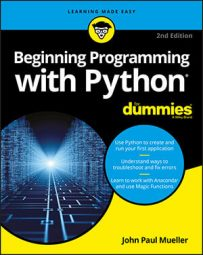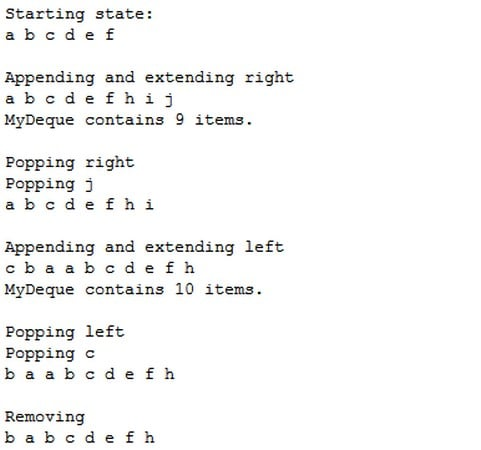##### Beginning Programming with Python For DummiesA `deque` is simply a `queue` where you can remove and add items from either end. In many languages, a `queue` or stack starts out as a `deque`. Specialized code serves to limit `deque` functionality to what is needed to perform a particular task.

When working with a `deque`, you need to think of the `deque` as a sort of horizontal line. Certain individual functions work with the left and right ends of the `deque` so that you can add and remove items from either side. The following steps help you create an example that demonstrates `deque` usage. This example also appears with the downloadable source code as `DequeData.py`.

1. Type the following code into Notebook — pressing Enter after each line. `import collections` `MyDeque = collections.deque("abcdef", 10)` `print("Starting state:")` `for Item in MyDeque:` `print(Item, end=" ")` `print("\r\n\r\nAppending and extending right")` `MyDeque.append("h")` `MyDeque.extend("ij")` `for Item in MyDeque:```` print(Item, end=" ")``` `.format(len(MyDeque)))` `print("\r\nPopping right")` `print("Popping {0}".format(MyDeque.pop()))` `for Item in MyDeque:` `print(Item, end=" ")` `print("\r\n\r\nAppending and extending left")` `MyDeque.appendleft("a")` `MyDeque.extendleft("bc")` `for Item in MyDeque:`? `print(Item, end=" ")` `print("\r\nMyDeque contains {0} items."` `.format(len(MyDeque)))` `print("\r\nPopping left")` `print("Popping {0}".format(MyDeque.popleft()))` `for Item in MyDeque:` `print(Item, end=" ")` `print("\r\n\r\nRemoving")` `MyDeque.remove("a")` `for Item in MyDeque:` `print(Item, end=" ")`

The implementation of `deque` is found in the `collections` package, so you need to import it into your code. When you create a `deque`, you can optionally specify a starting list of iterable items (items that can be accessed and processed as part of a loop structure) and a maximum size, as shown.

A `deque` differentiates between adding one item and adding a group of items. You use `append()` or `appendleft()` when adding a single item. The `extend()` and `extendleft()` functions let you add multiple items. You use the `pop()` or `popleft()` functions to remove one item at a time. The act of popping values returns the value popped, so the example prints the value onscreen. The `remove()` function is unique in that it always works from the left side and always removes the first instance of the requested data.

Unlike some other collections, a deque is fully iterable. This means that you can obtain a list of items by using a `for` loop whenever necessary.

2. Click Run Cell.

Python outputs the information (the screenshot shows only the output and none of the code).A deque provides the double-ended functionality and other features you’d expect.

Following the output listing closely is important. Notice how the size of the `deque` changes over time. After the application pops the j, the `deque` still contains eight items. When the application appends and extends from the left, it adds three more items. However, the resulting `deque` contains only ten items. When you exceed the maximum size of a `deque`, the extra data simply falls off the other end.